Friday, October 22, 2021
Home > Latest Announcement > NMTC 2016 Question Papers With Solutions Sub Junior Level

# NMTC 2016 Question Papers With Solutions Sub Junior Level# NMTC 2016 Question Papers with Solutions Sub Junior Level

NMTC is an acronym for National Mathematics Talent Contest. NMTC is conducted by AMTI (Association of Mathematics Teachers of India) to identify the talent of the students in Mathematics. In this post, NMTC 2016 Question Papers with Solutions Sub Junior Level is published.

This NMTC 2016 Question Papers with Solutions Sub Junior Level will help you understand what is the level of NMTC.

Part A: Instruction:

Only One of the choices A, B, C, D is correct. For each correct response, you get 1 mark. For each incorrect response, you lose 1/2 mark.

NMTC 2016 Paper For Sub Junior Level Ques No 1:

On the square ABCD , point E lies on the side AD and F lies on BC so that BE = EF = FD = 30 cm. The area of the square (in square cms) isOptions:

A. 300

B. 900

C. 800

D. None of these

Solution:NMTC 2016 Paper For Sub Junior Level Ques No 2:

If 2016 = 2x3y5z7u where x, y, z, u are non-negative integers, the value of x + y + 2016z + 3u is

Options:

A. 10

B. 11

C. 12

D. 13

Solution: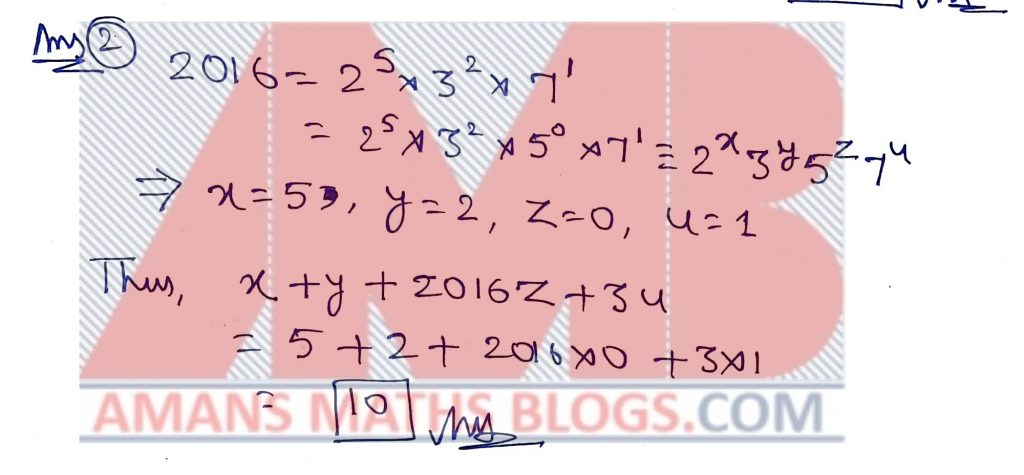NMTC 2016 Paper For Sub Junior Level Ques No 3:

A is the area of triangle of sides 25, 25 and 30. B is the area of a triangle of sides 25, 25 and 40. Then

Options:

A. A = B

B. A < B

C. A = 3B

D. A = 2B

Solution: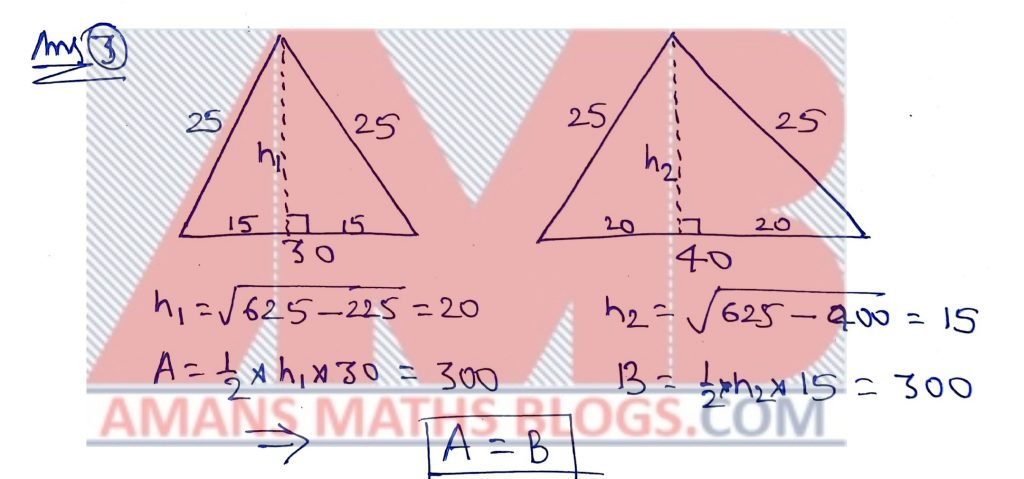NMTC 2016 Paper For Sub Junior Level Ques No 4:

A number on being divided by 5 leaves a remainder 2 and when divided by 7, leaves a remainder 4. The remainder when the same number is divided by 5 × 7 is

Options:

A. 20

B. 23

C. 32

D. None of these

Solution:NMTC 2016 Paper For Sub Junior Level Ques No 5:

The sum of three numbers is 204. If the ratio of the first to second is 2 : 3 and that of the second to third is 5 : 3. Then the second number is

Options:

A. 60

B. 65

C. 58

D. 90

Solution: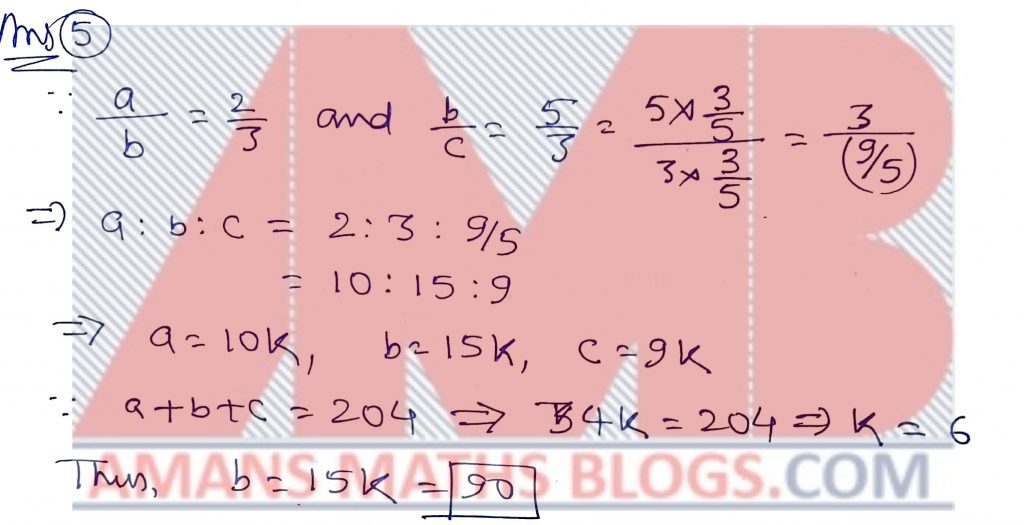NMTC 2016 Paper For Sub Junior Level Ques No 6:

Anirud goes to the vegetable market to buy onions. He carries money to buy 3 kg onions. But on reaching the market he finds that the price of onions has increased by 20%. If he buys onions for the money he has, the quantity he could buy is

Options:

A. 2.5 kg

B. 2.2 kg

C. 2 kg

D. 2.8 kg

Solution: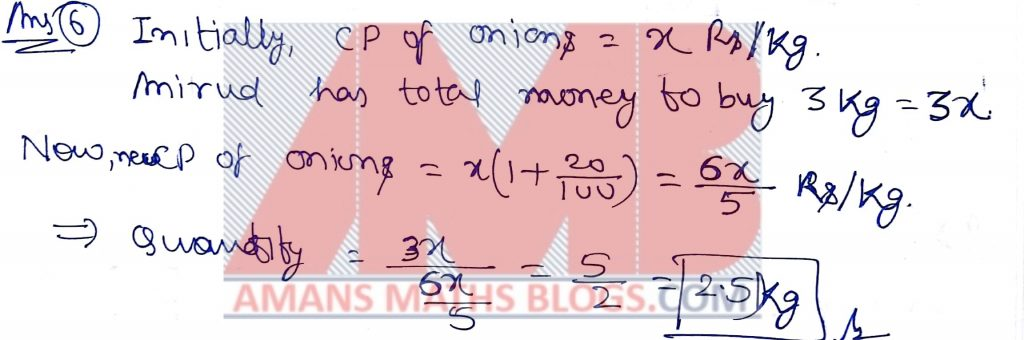NMTC 2016 Paper For Sub Junior Level Ques No 7:

Two runners cover the same distance at the rate of 15 km per hour and 16 km per hour respectively. The first runner took 16 minutes longer than the second to cover the distance. Then the distance (in km) is

Options:

A. 58

B. 64

C. 66

D. 73

Solution:NMTC 2016 Paper For Sub Junior Level Ques No 8:

The total surface area of a cube is 600 cm2. The length of its diagonal in cms is

Options:

A. 10

B. 30

C. 10D. 10Solution:

NMTC 2016 Paper For Sub Junior Level Ques No 9:

In the figure below the distance between any two horizontal or vertical dots is one unit. The area of the triangle shown isOptions:

A. 1/2

B. 1/3

C. 1/4

D. 1/6

Solution: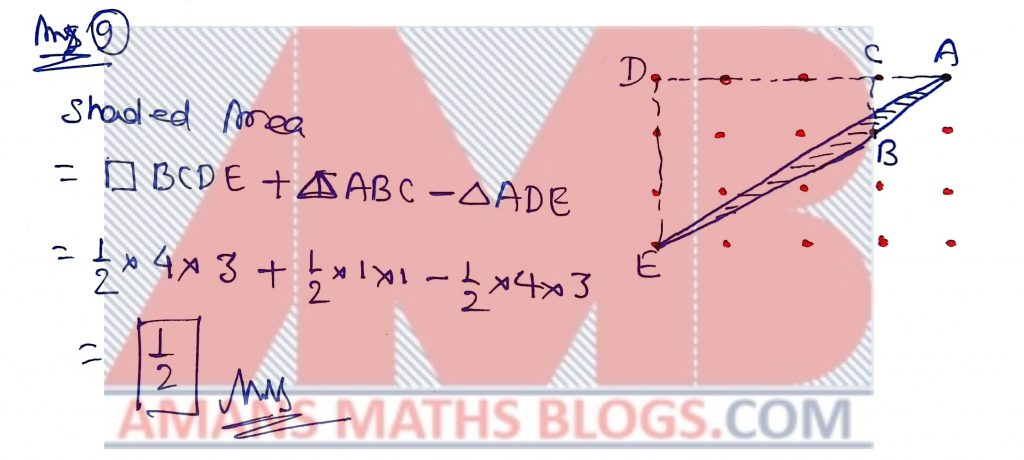NMTC 2016 Paper For Sub Junior Level Ques No 10:

The number of natural numbers less than 400 that are not divisible by 17 or 23 is

Options:

A. 290

B. 320

C. 360

D. 370

Solution:NMTC 2016 Paper For Sub Junior Level Ques No 11:

If a is a % of b and b is b % of c, where a is a positive real number, then the value of c is

Options:

A. 120

B. 200

C. 150

D. 100

Solution: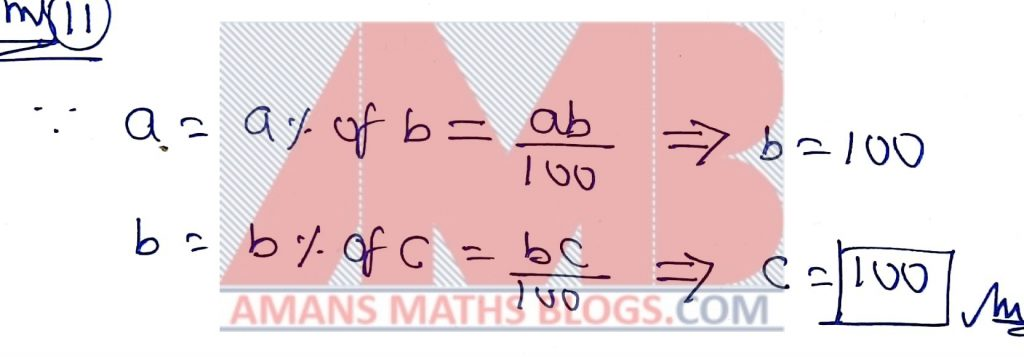NMTC 2016 Paper For Sub Junior Level Ques No 12:

The sum of the reciprocals of all the positive integers that divide 24 is

Options:

A. 7/2

B. 1/2

C. 5/2

D. 3/2

Solution:NMTC 2016 Paper For Sub Junior Level Ques No 13:

N is a positive integer and p, q are primes. If N = pq and , the value of N is

Options:

A. 6

B. 7

C. 8

D. 9

Solution: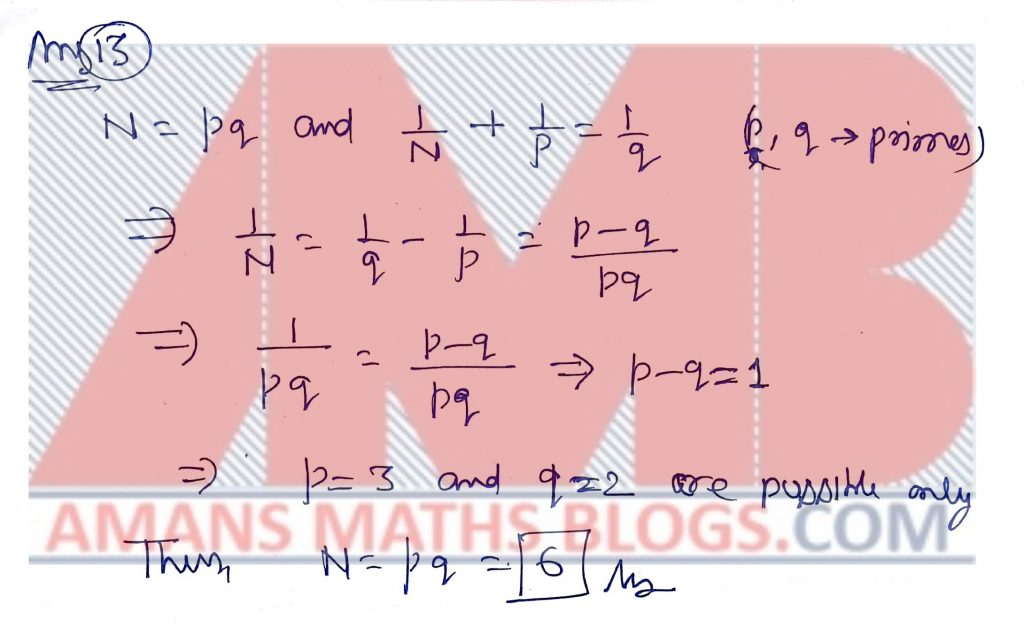NMTC 2016 Paper For Sub Junior Level Ques No 14:

The number of two digit numbers that leave a remainder 1 when divided by 4 is

Options:

A. 20

B. 21

C. 22

D. 23

Solution: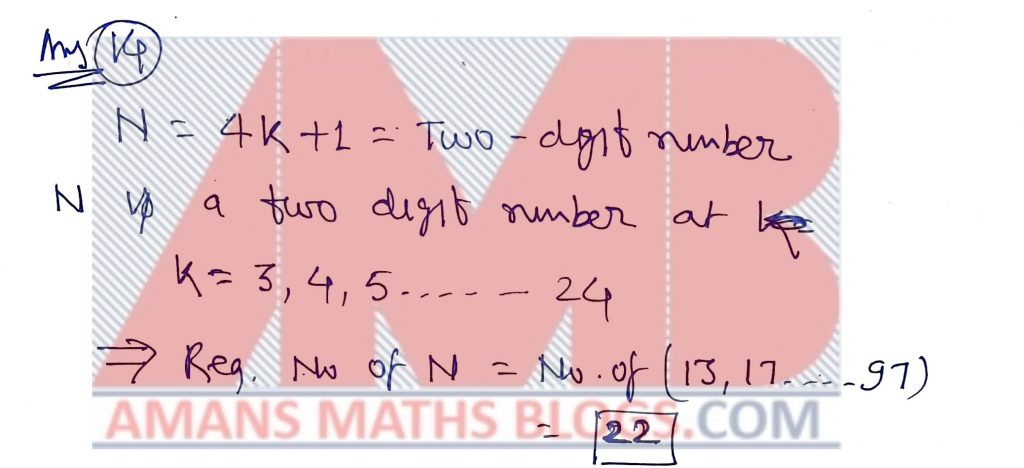NMTC 2016 Paper For Sub Junior Level Ques No 15:

If (a + b)2 + (b + c)2 + (c + d)2 = 4(ab + bc + cd) , then

Options:

A. a + b or b + c or c + d must zero

B. Two of a, b, c, d are zero and other two non-zero

C. a = b = x = d

D. None of these

Solution:Part B: Instruction:

Write the correct answer in the space provided in the responsive sheet. For each correct response, you get 1 mark. For each incorrect response, you lose 1/4 mark.

NMTC 2016 Paper For Sub Junior Level Ques No 16:

A race horse eats (3a + 2b) bags of oats every week. The number of weeks in which it can eat (12a2 − 7ab − 10b2) bags of oats is ____.

Solution:NMTC 2016 Paper For Sub Junior Level Ques No 17:

Choose 4 digits a, b, c, d from {2, 0, 1, 6} and form the number (10a + b)10c+d. For example, if a = 2, b = 0, c = 1, d = 6, we will get 2016. For all such choices of a, b, c, d the number of distinct numbers that will be formed is

Solution: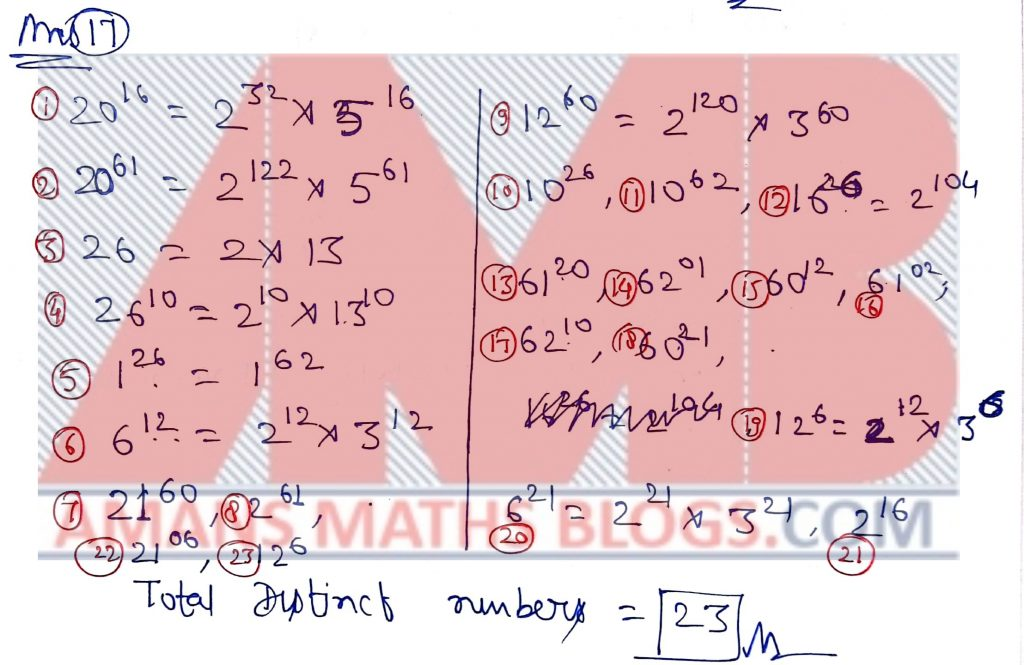NMTC 2016 Paper For Sub Junior Level Ques No 18:

A fraction F becomes 1/2 when its denominator is increased by 4 and becomes 1/3 when its numerator is decreased by 5. Then F equals _____.

Solution:NMTC 2016 Paper For Sub Junior Level Ques No 19:

The average of 5 consecutive positive integers starting with m is n. Then the average of 5 consecutive integers starting with n is (in terms of m ) is

Solution:NMTC 2016 Paper For Sub Junior Level Ques No 20:

Two boys came to Mahadevan and asked his age. Mahadevan told, “Delete all the vowels and repeated letters from my name. Find the numerical value of the remaining letters (for example, D has value 4, G has 7 etc.). Add all of them. Find the number got by interchanging its digits. Add both the numbers. That is my age”. One boy ran away. The other boy calculated correctly. The age of Mahadevan is _____.

Solution: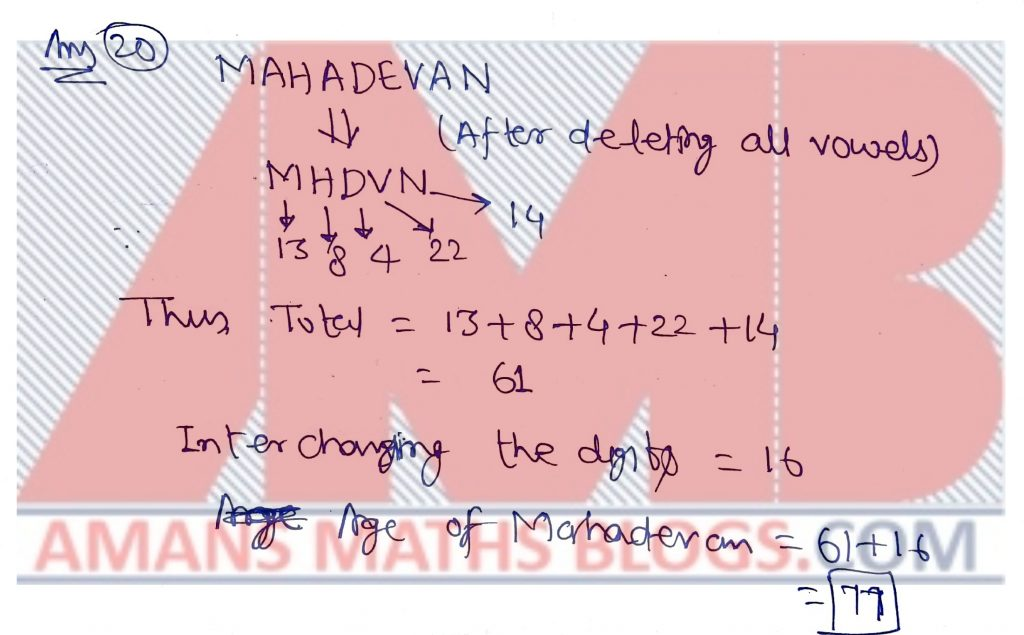NMTC 2016 Paper For Sub Junior Level Ques No 21:

If A =, B =, C =, then the integral part of (A + C)/B is ____.

Solution:NMTC 2016 Paper For Sub Junior Level Ques No 22:

A six-digit number is formed using the digits 1, 1, 2, 2, 3, 3. The number of 6-digit numbers in which the 1s are separated by one digit, 2s are separated by two digits and 3s are separated by 3 digits is ____.

Solution: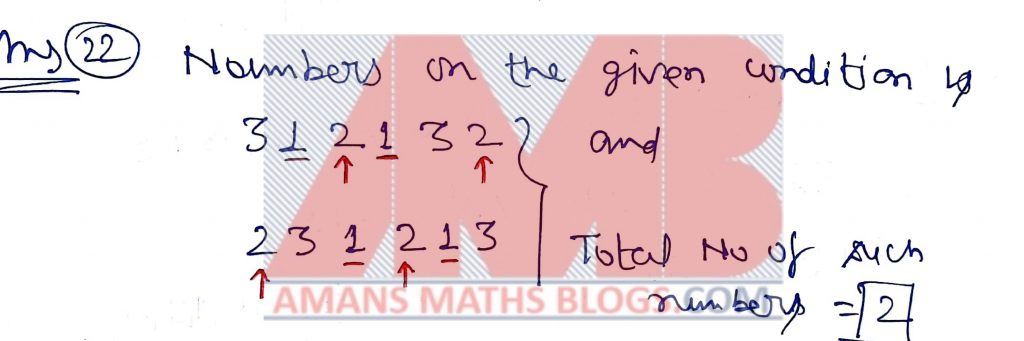NMTC 2016 Paper For Sub Junior Level Ques No 23:

In the addition shown below, P, I, U are digits. The value of U is__.

PI + PI + PI + PI = UP

Solution:NMTC 2016 Paper For Sub Junior Level Ques No 24:

There are four cows, eight hen, a fish, a crow, a girl and a boy in a garden. Outside the garden there is one dog, a peacock and some cats. The number of legs of all of them inside the garden is equal that outside the garden. The number of cats is ____.

Solution: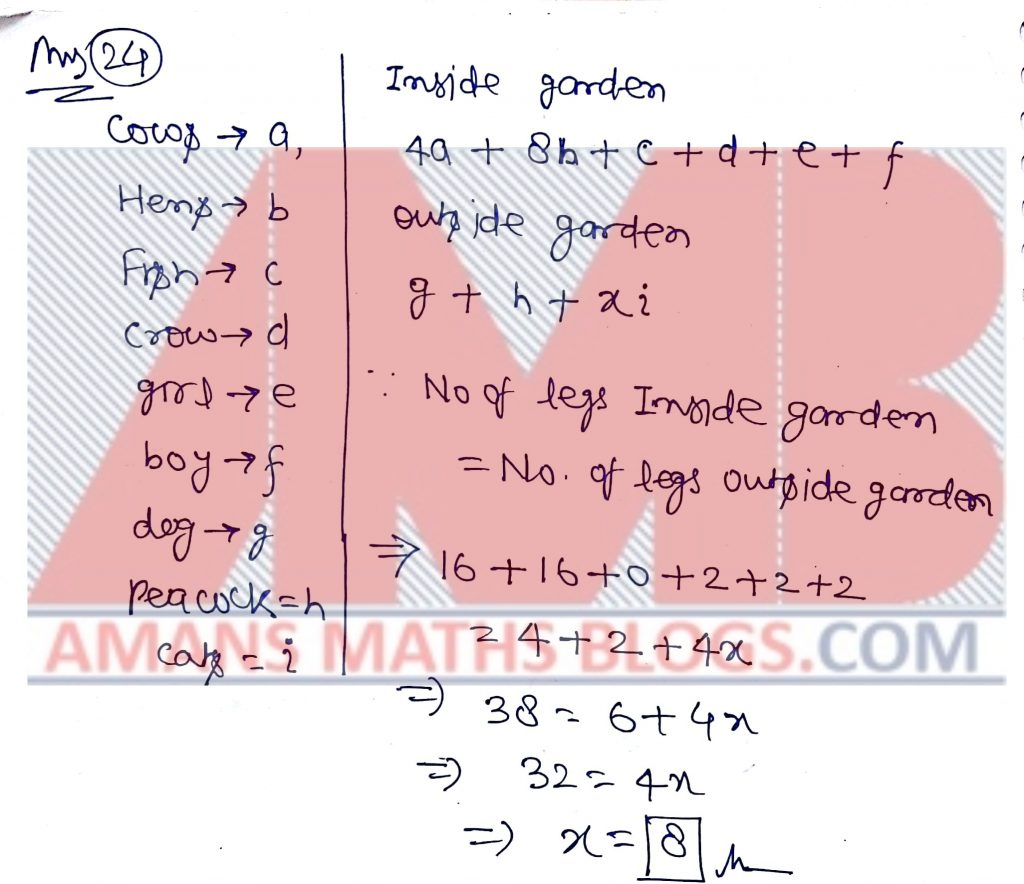NMTC 2016 Paper For Sub Junior Level Ques No 25:

Two sides of a triangle are 8 cm and 5 cm. The length of the third side in cms is also an integer. The number of such triangles is _____.

Solution: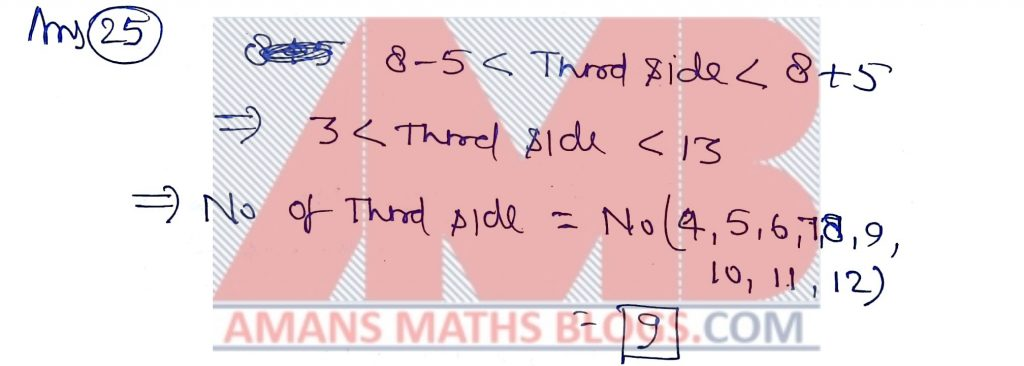NMTC 2016 Paper For Sub Junior Level Ques No 26:

If a2 − a − 10 = 0 , then (a + 1)(a + 2)(a − 4) is _____.

Solution: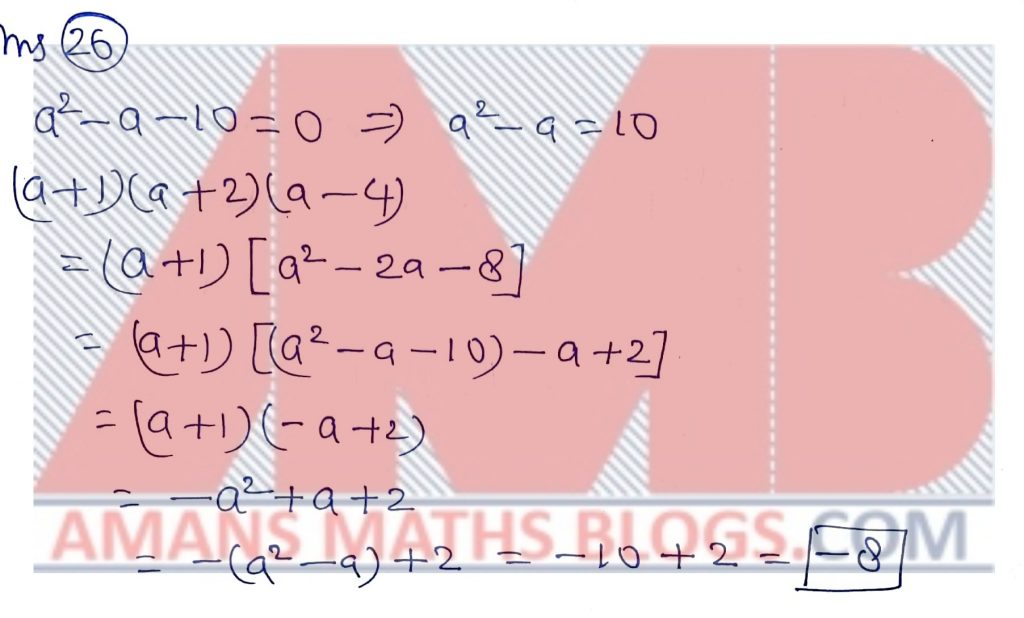NMTC 2016 Paper For Sub Junior Level Ques No 27:

There are 5 points on the circumference of a circle. The number of chords which can be drawn joining them is _____.

Solution: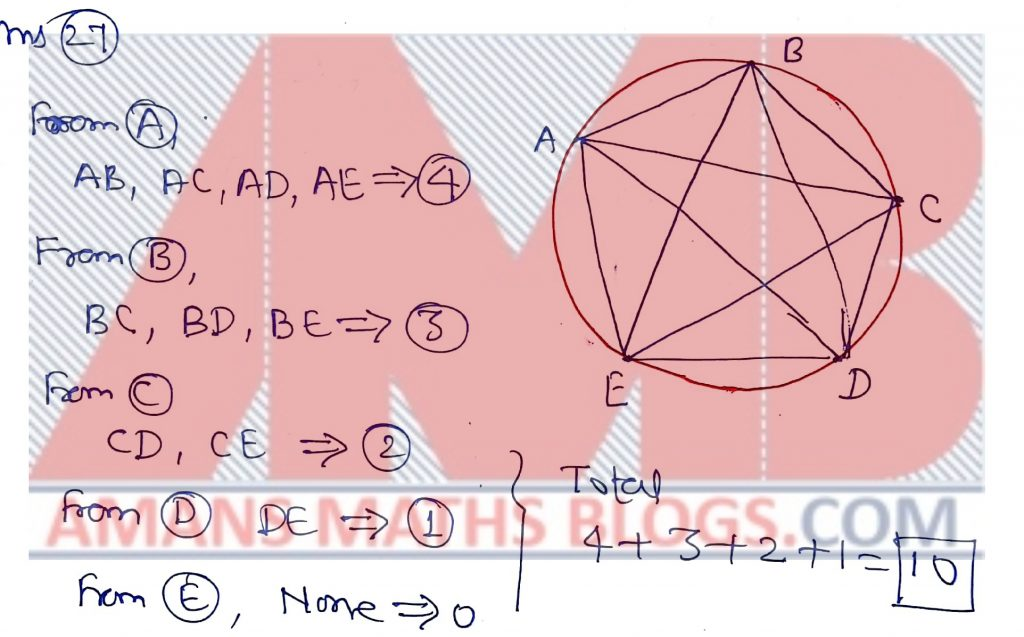NMTC 2016 Paper For Sub Junior Level Ques No 28:

Each side of an equilateral triangle is 3 cm longer than each side of a square. The total perimeter of the square and the triangle is 51 cm. Then the side of the triangle in cms is _____.

Solution:NMTC 2016 Paper For Sub Junior Level Ques No 29:

The largest three digit number that is a multiple of 3 and 5 is _____.

Solution: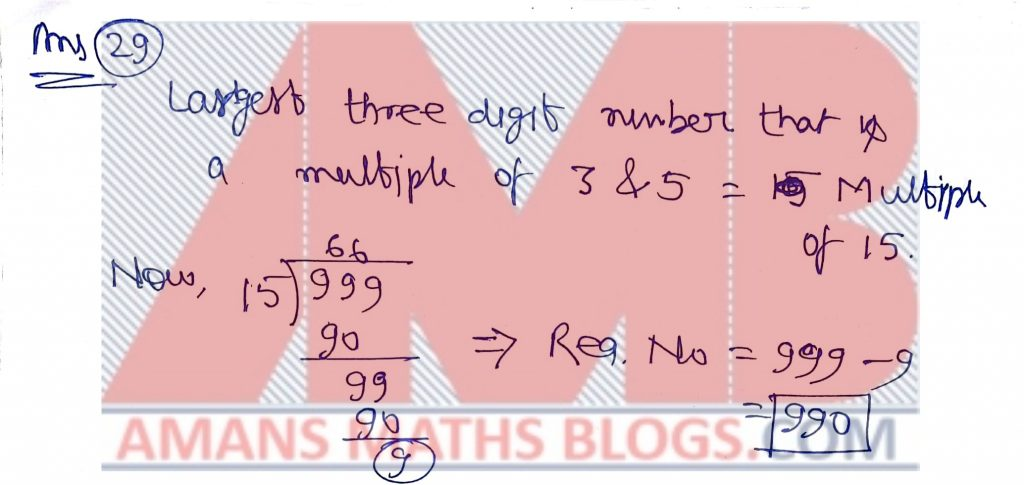NMTC 2016 Paper For Sub Junior Level Ques No 30:

Consider the sequence 0, 6, 24, 60, 120, . . . .The 6th term of this sequence is _____.

Solution: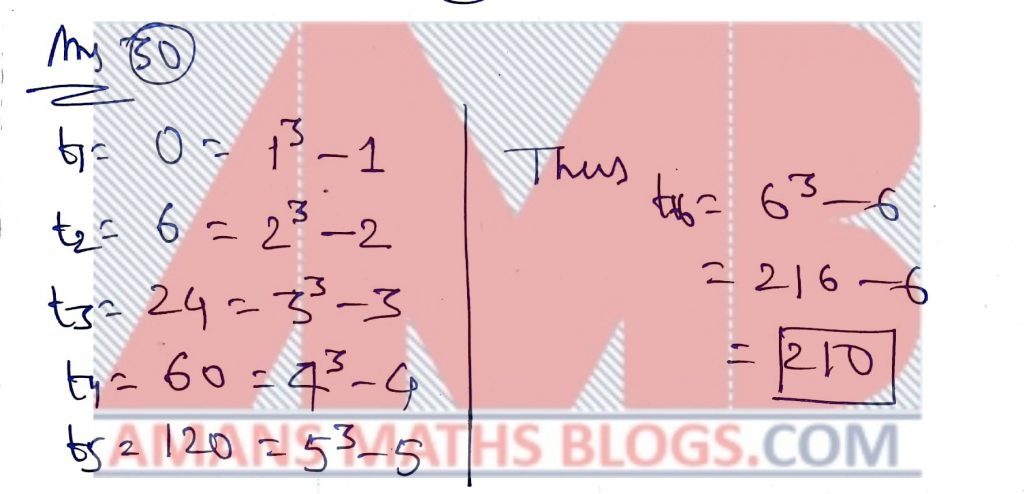AMBiPi
error: Content is protected !!